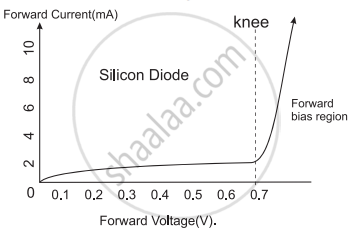# Answer in detail. Explain the I-V characteristic of a forward-biased junction diode. - Physics

Explain the I-V characteristic of a forward-biased junction diode.

#### Solution

1. Figure given below shows the I-V characteristic of a forward-biased diode.Forward biased characteristic of a diode
2. When connected in forward bias mode, initially, the current through the diode is very low and then there is a sudden rise in the current.
3. The point at which currently rises sharply is shown as the ‘knee’ point on the I-V characteristic curve.
4. The corresponding voltage is called the knee voltage. It is about 0.7 V for silicon and 0.3 V for germanium.
5. A diode effectively becomes a short circuit above this knee point and can conduct a very large current.
6. To limit the current flowing through the diode, resistors are used in series with the diode.
7. If the current through a diode exceeds the specified value, the diode can heat up due to the Joule’s heating and this may result in its physical damage.
Concept: A p-n Junction Diode
Is there an error in this question or solution?

#### APPEARS IN

Balbharati Physics 11th Standard Maharashtra State Board
Chapter 14 Semiconductors
Exercises | Q 3. (iv) | Page 256